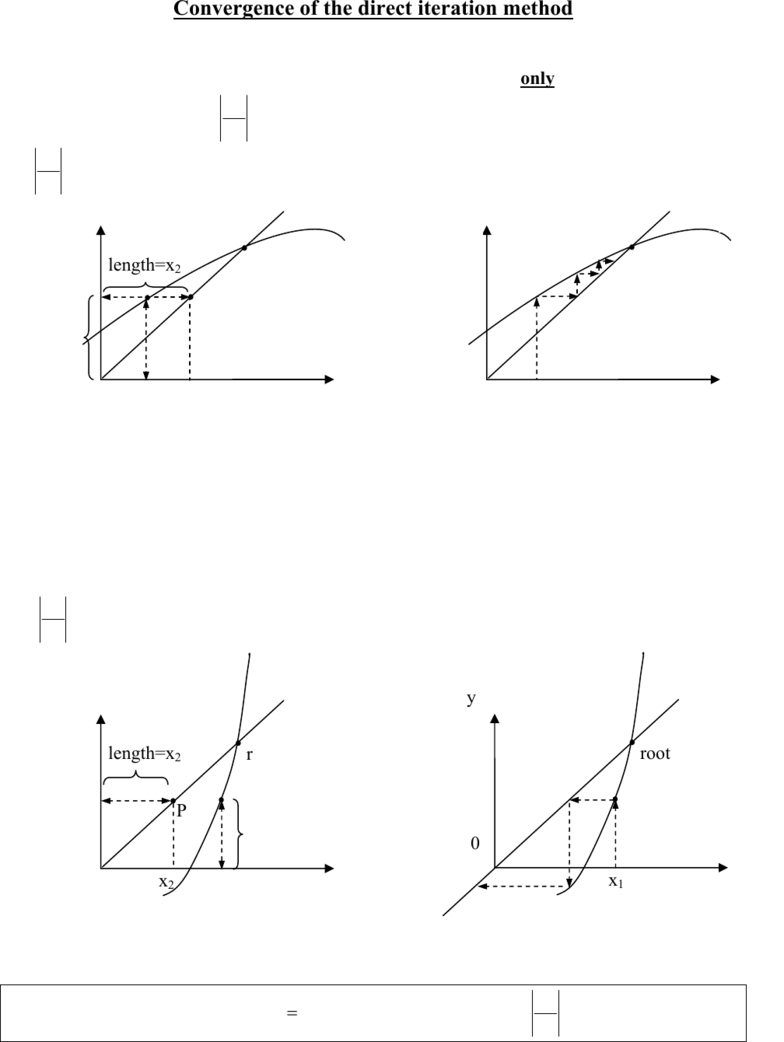Textbook Notes (280,000)
CA (160,000)
UW (6,000)
ME (1)
ME303 (1)
Chapter

Direct Iteration Method

Department
Mechanical Engineering
Course Code
ME303
Professor
Serhiy Yarusevych

Page:
of 1Convergence of the direct iteration method
The )(xgx = rearrangement that we choose can have a strong effect on convergence of the
iteration. We can show graphically that the iteration will converge only if the function )(xg on
the right hand side satisfies 1<
dx
dg near the root.
(i) 1<
dx
dg
Calculations:
1) guess x1
2) compute g(x1)
3) x2= g(x1), i.e., the length corresponding to x2 equals to the length given by g(x1), as shown on
the sketch above. Thus, the point P(x=xnew, y=g(xold) ) falls on the line y=x. In this case,
repeating the process moves P along y=x towards the root and the iterations eventually converge,
as shown on the sketch.
(ii) 1
dx
dg
Calculations here are the same as above. In this case, however, repeating the process moves P
along y=x away from the root and the iterations do not converge on the root, as shown in the
sketch.
The Rule: always choose an )(xgx
=
form corresponding to 1<
dx
dg near the root.
x2
length=x2
x
y
y=g(x)
P
root
0
g(x1)
y=x (slope=1)
x1 x
y
root
0
x1
y=g(x)
y=x
length=x2
x
y
x1
y=g(x)
x2
P
root
0
g(x1)
y=x (slope=1) y y=x
root
x1
y=g(x)